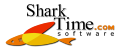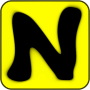What is Sharky Neural Network for?
For fun, for education, for better understanding of neural networks.
Is Sharky Neural Network free?
Yes, Sharky Neural Network is indeed free, i.e we do not charge any money for it. You can use it both for private and commercial use. We would be very glad, if you will inform us where and how you are using this software.
What is neural network?
An artificial neural network (ANN), usually called "neural network" (NN), is a fascinating mathematical technique which conducts complex computations. You can find more information here.
What does this neural network recognize?
This neural network classifies 2D points into two different classes (yellow and blue). It does not classify shapes! The shapes you can see are a visualization of these points classification. If you ask what it recognizes, then we may say, that it recognizes if a 2D point (vector described by two real values) is blue or yellow.
Why is this network 2:...:2 structure?
The former "2" digit, means 2 inputs, because each 2D point has two values - x and y. The latter "2" digit, means 2 outputs, because this network classifies into 2 different classes (yellow, blue). In that way, this network is open for classification into more classes. More classes feature will be added in a future.
How is single neuron build?
Each neuron has bias and is using bipolar sigmoid activation function (`f(x)=2/(1+e-βx)-1`).
Can I add my own points?
Yes, you can add, delete, load or save points. Use Ctrl + Left Click for adding many points at once (spray).
Can I define my own shapes?
No. You may setup points in a custom shape and NN will learn it. However, "verification" and "generate points" won't work properly.
How did you create "N" logo?
You may do it yourself. Just open points (Ctrl + O) from path below, choose network structure, setup learning parameters and press "Learn" button.
```C:\Program Files\Sharky Neural Network\points\N.points
```